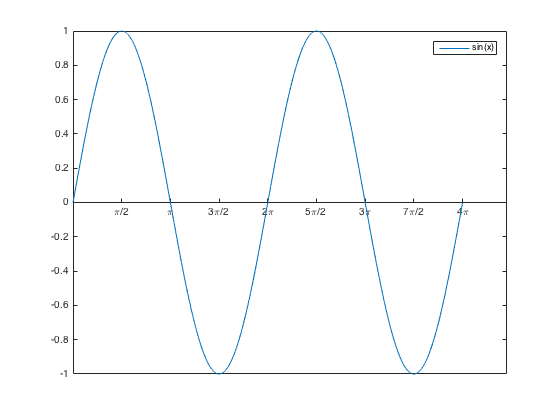Categories

# Non-linearity of Sine

While at a meeting yesterday the following question came up due to a student “Why isn’t $$\mathrm{sin}(a+b) = \mathrm{sin}(a) + \mathrm{sin}(b) \sin$$“. My immediate response would be to say “because $$\sin(x)$$ isn’t a linear function”, but this isn’t a terribly satisfactory response since the likelihood is that the student doesn’t have a deep understanding of the difference between linear and non-linear functions.

On reflection I think the best explanation is to ask the student to sketch the graph of $$\sin(x)$$ and ask them to consider $$\sin(a+b)$$ using the graph.It should become clear from the graph that $$\mathrm{sin}(a+b)$$ can only be equal to $$\mathrm{sin}(a) + \mathrm{sin}(b)$$ when either one of $$a$$ or $$b$$ is $$360^\circ$$ (in fact any multiplicity of the periodicity of $$\sin(x)$$.

Another approach could be to ask them to consider a sequence of non-linear functions such as $$f(x)=x^2, f(x) = x^3+3, f(x) = 2^x+6$$ and ask them to compute $$f(a),f(b),f(a+b)$$. This I hope would get rid of the expectation that f(a+b) = f(a)+f(b). The geometric proofs of the true formulae for $$\sin(a+b)$$ could be a nice way to close the discussion.

I wonder if this is a consequence of an over-reliance on linear functions for examples of substitution etc in lower school.